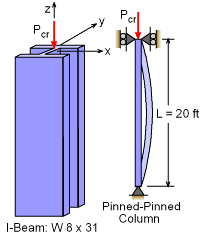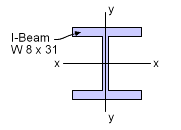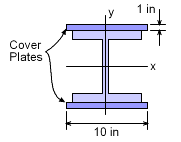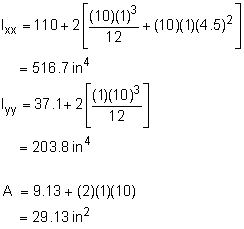Ch 9. Columns Multimedia Engineering Mechanics BasicColumns FixedColumns EccentricLoads
 Chapter 1. Stress/Strain 2. Torsion 3. Beam Shr/Moment 4. Beam Stresses 5. Beam Deflections 6. Beam-Advanced 7. Stress Analysis 8. Strain Analysis 9. Columns Appendix Basic Math Units Basic Equations Sections Material Properties Structural Shapes Beam Equations Search eBooks Dynamics Fluids Math Mechanics Statics Thermodynamics Author(s): Kurt Gramoll ©Kurt GramollMECHANICS - EXAMPLE ExampleA 20 foot high steel column is constructed from an I-beam W8×31. In order to strengthen the section, the engineer decided to cover each flange with a 10 in by 1 in steel plate as shown in the diagram. What is the increase in critical load capacity of the new column? Assume the steel modulus of elasticity, Esteel, is 29,000 ksi and the yield stress, σyld, is 36 ksi. SolutionBasic W8x31 I-Beam A simply supported column (pinned at each end) will buckle around the axis with the lowest moment of inertia. The moment of inertia of a standard W8×31 can be found in the Structure Shapes appendix as,      Ixx = 110 in4      Iyy = 37.1 in4      A = 9.13 in2 The lowest critical load for this I-beam is around the y axis,      Pcr = π2 EIyy / L2            = π2 (29,000 ksi)(37.1 in4) / {(20 ft) (12)}2            = 184.4 kip The critical stress,       σcr = Pcr / A            = 184.4 / 9.13            = 20.20 ksi This is less than the yield stress of 36 ksi so application of Euler's equation is appropriate.W8x31 I-Beam with Cover Plates To increase the critical load of the section, the I-Beam is covered with a plate 10 in × 1 in. For this new section, the moment of inertia and area are,The improved section Iyy still has a lower value than Ixx. So, it will buckle around y axis. Critical load with the cover plates is      Pcr = π2 EIyy / L2            = π2 (29,000 ksi)(203.8 in4) / {(20 ft)(12)}2            = 1,013 kip The critical stress,       σcr = Pcr / A            = 1013 / 29.13            = 34.78 ksi This stress is still below the yield stress so Euler's equation is still appropriate. The increase in critical load capacity,      δPcr = (1013 - 184.4) kip              = 828.6 kip or nearly 450% increase.

Practice Homework and Test problems now available in the 'Eng Mechanics' mobile app
Includes over 400 problems with complete detailed solutions.
Available now at the Google Play Store and Apple App Store.